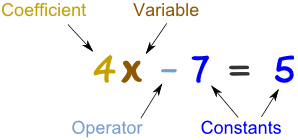How do you solve -9 + 8k = 7 + 4k?

Jan 5, 2017

k=4

Explanation:

Okay so first we have to keep all the Like Terms on a separate side.

I will include the Google Definition of Like Terms Down Below:
Google Definition: Like terms are terms that contain the same variables raised to the same power.

$- 9 + 8 k = 7 + 4 k$?

The like terms in this case are 8k and 4k. They both end in k therefore they are like terms.

-9 and 7 are like terms because they have no variable.

What is a variable? Maybe this image below will helpTo summarize, the coefficent is what is with the variable such as 4. It is the number before the letter basically.

Now lets continue, to our orginal problem.

$- 9 + 8 k = 7 + 4 k$?

In my opinion, I like all my like terms with an alphabet on my left and the numbers without the variable (letters) on my right.

So let's do what i just said...

$8 k - 4 k = 7 + 9$?

But now why are the symbols changed?? Positive 4k is -4k and Negative 9 is now 9!!

The symbols are changed because we are trying to cancel out the number one side so we could make it 0 and bring it to the other side. But to do that we have to use the opposite sign.

For example if a number is 4 then to cancel it out to equal 0 the number to cancel it out would be -4.

Last example, try it on your own (cover up the answer below)
If the number is -7893 to cancel it out you have to add the number
7893.

Get the hang of it? Now back to the original problem.

$8 k - 4 k = 7 + 9$?

Now just subtract/add the like terms together!

$4 k = 16$

This is the tricky part; we have to make sure the variable (the letter) is ALL ALONE. I know right poor little alphabet. But, sometimes you just do what'd you got to do.

Now remember what we did in the beginning to make 4k to come onto the other side we had to subtract each side to cancel 4k on one side out to make it come on the other side.

Same here divide both sides by 4. MAKE SURE YOU DON'T DIVIDE BY THE OPPOSITE NUMBER. Although it sounds strange, it's not it's just how math works.

Now divide both sides by 4 and you will get.

$k = 4$

Happy Learning!